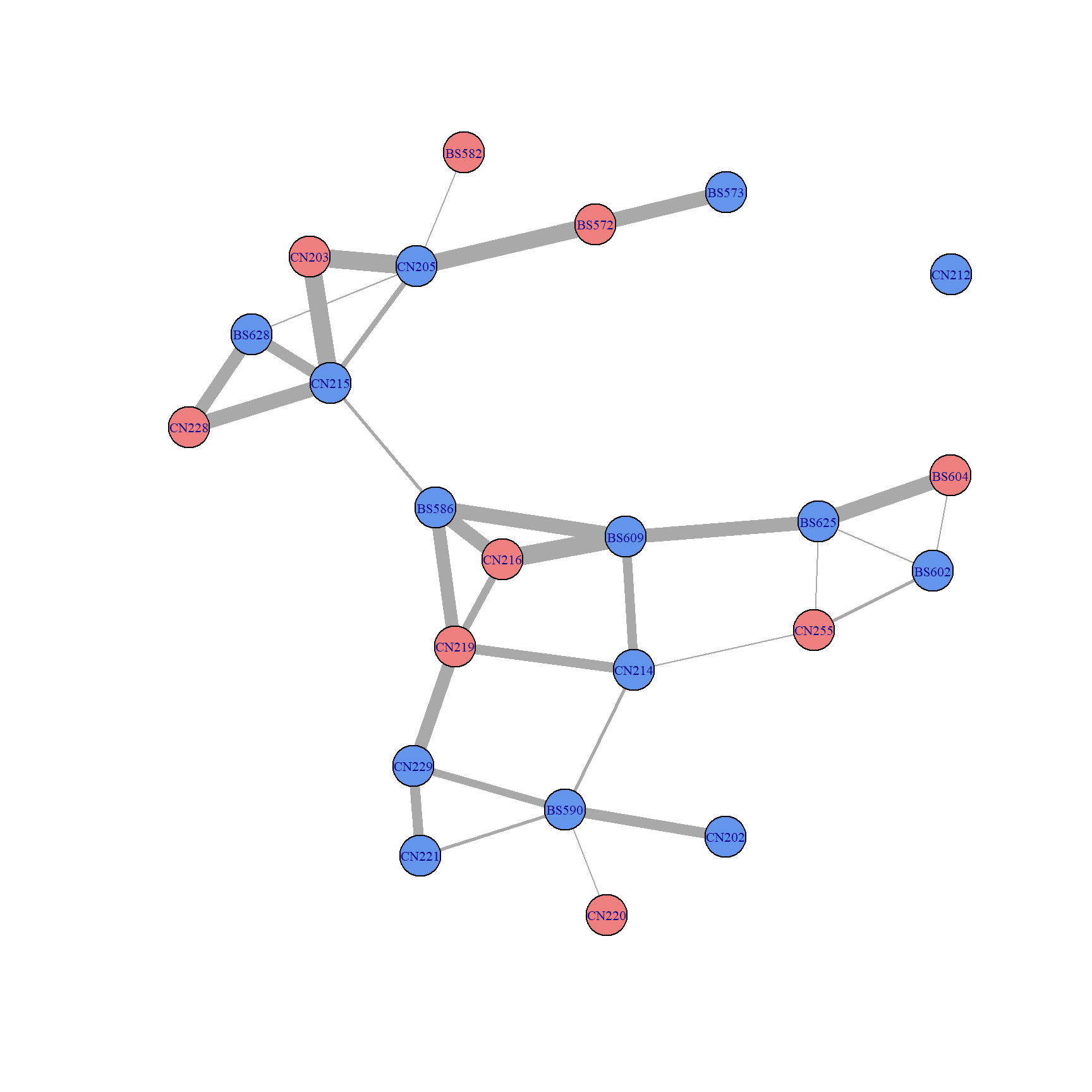## Example - tortoise social relationships

Now we’ll look at an example where we use movement data to create a network of social relationships. In this example, we’ll be working through the process of manipulating and processing data in order to put it in a format amenable to graphs.

The data we’re using comes from GPS tracked tortoises. We’ll be looking at the data for tortoises at one site (“SouthPah”) for 2018. The GPS trackers record a tortoise’s location once every hour. What we want to do in this example is make a network based on social interactions - we want a graph where the nodes are tortoises and the edges indicate that two tortoises had at least one interaction in 2018 (4/25 - 10/11). First, let’s load the data and take a look at it.

#load the data - this loads variables called "torts" and "tort_info"
#'torts' contains the tortoise tracking data for the SouthPah site in 2018. There were 23 tortoises
#that were GPS-tracked at this site. A location was recorded once every hour.

#'tort_info' contains basic information about each tortoise

#check out the data (I'm only displaying the columns that are relevant for this example)
head(as.data.frame(torts)[,c("tort", "datetime", "x", "y")])
##        tort            datetime        x       y
## 78402 BS572 2018-04-25 07:25:41 644799.6 3930122
## 78403 BS572 2018-04-25 08:27:50 644761.4 3930093
## 78404 BS572 2018-04-25 09:32:13 644675.3 3930066
## 78405 BS572 2018-04-25 10:32:51 644626.2 3930102
## 78406 BS572 2018-04-25 11:33:32 644629.7 3930103
## 78408 BS572 2018-04-25 12:34:23 644629.8 3930100
head(tort_info)
##    Tortoise NumEntries TotalTimeDays  FirstDate   LastDate NumBurrows
## 12    BS572       3613      169.1793 2018-04-25 2018-10-11         12
## 13    BS573       3036      141.0630 2018-04-25 2018-09-13         12
## 14    BS582       3007      169.0548 2018-04-25 2018-10-11         10
## 15    BS586       2901      141.0705 2018-05-23 2018-10-11         15
## 16    BS590       3066      140.9097 2018-05-23 2018-10-11         12
## 17    BS602       2527      113.9924 2018-06-19 2018-10-11          9
##        Site LikelySEX LastMCL Site_tort_info
## 12 SouthPah         F     234       Southpah
## 13 SouthPah         M     254       Southpah
## 14 SouthPah         F     229       Southpah
## 15 SouthPah         M     280       Southpah
## 16 SouthPah         M     280       Southpah
## 17 SouthPah         M     218       Southpah
rownames(tort_info) = tort_info$Tortoise #plot the data, making each tortoise a different color unique_torts = unique(torts$tort) #get the unique tortoise names
tort_cols = rainbow(length(unique_torts)) #get a different color for each tortoise
names(tort_cols) = unique_torts #assign the names of the tortoises to our vector of colors
plot(torts, col=tort_cols[torts$tort], cex = .5, pch=16) #plot the data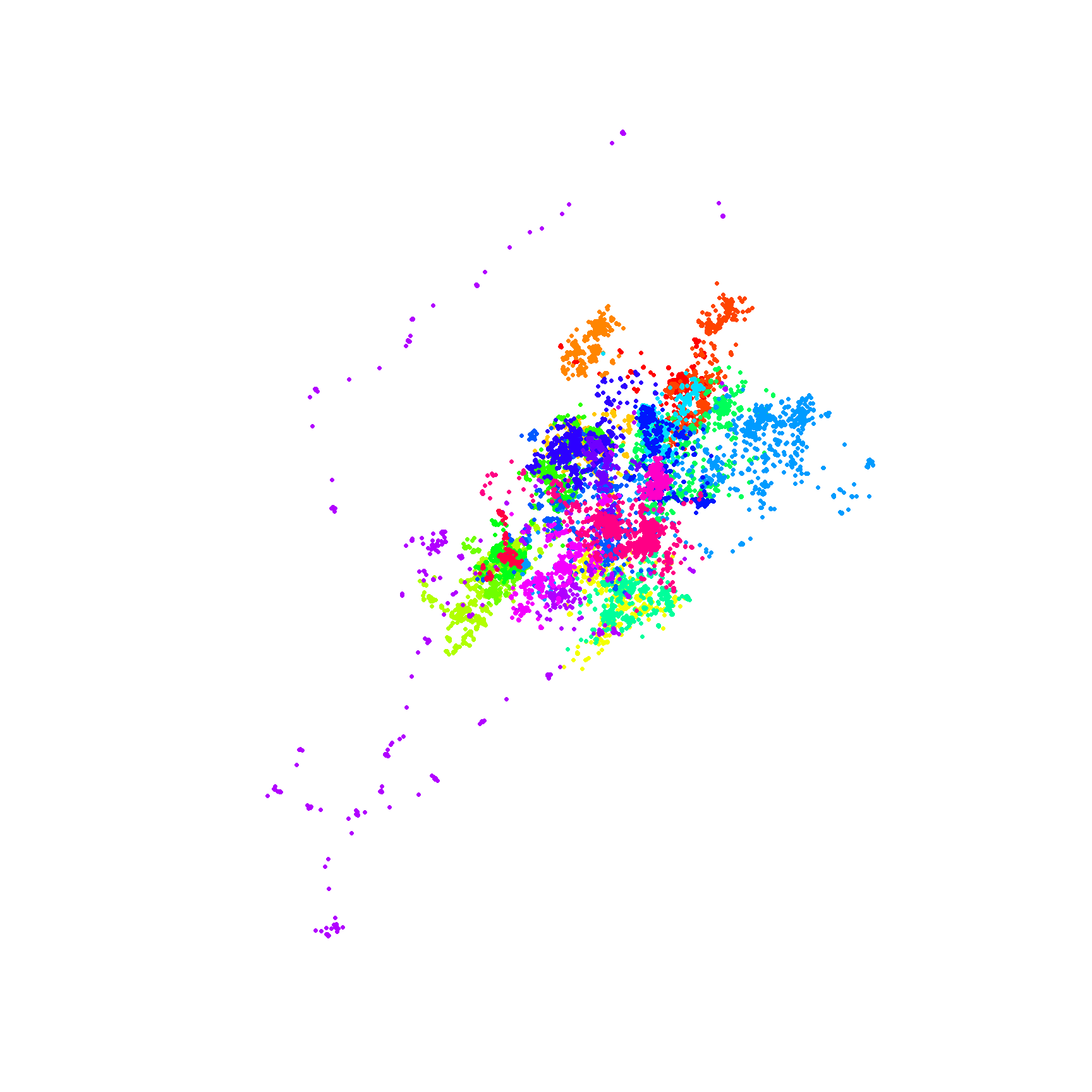### Step 1: Standardize the times To make a graph of the social interactions, we need to be able to detect when two tortoises are close to each other, so we’ll calculate the distances between tortoises at all time steps. However, there’s a problem - the times don’t line up. For example, one tortoise may have a fix at 10:17 and another may have a fix at 10:43. To avoid this problem, we’ll standardize the times - in this case, both times will be set to 10:30. This will introduce some error, but we should still be able to use this to make inferences about the tortoises’ social interactions. This makes use of some functions that I’ve already written - we won’t go over these, as they’re not relevant to this example. #get a vector of times that represents the times we want to standardize our data to - #use the 'adj_path_get_datetimes()' function that I wrote previously new_times = adj_path_get_datetimes(torts$datetime) #this function creates a vector times - these are the times we'll standardize our data to

#now we'll use the 'adj_path_times()' function to standardize the times for each tortoise
tort_adj_list = lapply(1:length(unique_torts), function(i){ #loop over the tortoise names, and standardize the times of the fixes
this_tort = torts[torts$tort == unique_torts[i],] #get the data for only this tortoise tort_adj = adj_path_times(pts = this_tort@coords, #standardize the times using 'new_times' as the reference set of times pts_times = this_tort$datetime,
new_times = new_times,
time_gap_limit = 72)
})
#tort_adj_list is a list of data frames. Give each data frame the name of the corresponding tortoise
head(tort_adj_list[])
##   actual_id id            datetime type  x  y
## 2        -1  1 2018-04-25 00:30:00    0 NA NA
## 3        -1  2 2018-04-25 01:30:00    0 NA NA
## 4        -1  3 2018-04-25 02:30:00    0 NA NA
## 5        -1  4 2018-04-25 03:30:00    0 NA NA
## 6        -1  5 2018-04-25 04:30:00    0 NA NA
## 7        -1  6 2018-04-25 05:30:00    0 NA NA

### Step 2: Calculate the distances at each time step.

Now that we have the times standardized, we can calculate the distance between tortoises at each time step. We’ll do this by calculating a distance matrix at each time step - this will tell us the distances between all pairs of tortoises

#get the distance matrix for all tortoises at each time
all_dists = lapply(1:length(new_times), function(i){ #loop over the vector of reference times (the times we standardized our data to)
return(tort_adj_list[[j]][i,c("x", "y")]) #for each tortoise, grab the 'i'th location
})
coords = do.call(rbind, coords_list) #rbind the locations together to get a data frame of the locations of each tortoise
rownames(coords) = names(tort_adj_list) #assign the tortoise names as row names
dist_mat = as.matrix(dist(coords)) #get the distance matrix between all tortoises at this moment in time
diag(dist_mat) = NA #get rid of the diagonal (this will always be 0 - the diagonal gives the distance between a tortoise and itself.)
dist_mat[upper.tri(dist_mat)] = NA #get rid of the upper triangle (this is the same as the lower triangle)
return(dist_mat)
})
all_dists[][14:17,1:5]
##           BS572     BS573     BS582     BS586     BS590
## CN212  452.2037  360.0633 1199.6542 1238.5734 1459.1895
## CN214 1187.6784 1081.7976 1413.9938  687.1740  164.2659
## CN215  740.6569  585.9494 1290.5514  887.2567  804.4556
## CN216 1034.2068 1024.5905  869.9438  130.0217  759.4366

### Step 3: Determine which pairs of tortoises interacted with each other

We now have the distance matrices for each time step. Now we’ll use this to find pairs of tortoises that were within 15m at some point in time. To do this, we’ll look at the distances between each pair of tortoises and keep track of the number of timestamps where they were within 15m - if this value is 1 or more, we’ll say that they interacted at some point. And the larger the number, the longer the tortoises spent together, so this gives us an idea of the strength of the relationship.

The type of calculation we want to do can be easily performed on rasters using the ‘raster’ package, so we’ll convert the list of distance matrices into a RasterBrick (essentially a bunch of rasters “stacked on top of each other). Then we can easily”drill down" through all of the distance matrices, looking at the distances between a given pair of tortoises, and counting how many times there’s a value less than 15.

rast_list = lapply(all_dists, raster) #convert each distance matrix into a raster
dists_rast = raster::brick(rast_list) #combine them to form a RasterBrick

#now for each cell we'll count the number of times the value is 15 or less.
n_close_rast = calc(dists_rast, function(vals_i){
if(all(is.na(vals_i))){
return(NA)
} else {
return(sum(vals_i < 15, na.rm=TRUE)) #return the number of TRUEs that result from 'vals_i < 15'
}
})
n_close = t(as.matrix(n_close_rast)) #convert the raster back to a matrix

rownames(n_close) = rownames(all_dists[]) #the row and column names don't transfer to the raster, so reassign them
colnames(n_close) = colnames(all_dists[])
n_close[5:9,9:13]
##       BS625 BS628 CN202 CN203 CN205
## BS590     0     0    22     0     0
## BS602     1     0     0     0     0
## BS604    92     0     0     0     0
## BS609    57     0     0     0     0
## BS625    NA     0     0     0     0

### Step 4: Use what we’ve done so far to create a graph

Now we know which tortoises have ‘interacted’ (at least by our definition), so we’ll use that to make a graph - we’ll make the tortoises the vertices and draw edges between tortoises that interacted with each other.

#use the 'melt' function to reformat our matrix to a data frame
n_close_df = reshape2::melt(n_close)

#We only want to make a graph with the pairs that were close to each other at some point in time (1 or more moments in time where the pair was less than 15m apart)
n_close_df_nz = n_close_df[n_close_df$value > 0 & !is.na(n_close_df$value),]
head(n_close_df_nz)
##      Var1  Var2 value
## 24  BS572 BS573    95
## 144 BS602 BS604     1
## 165 BS586 BS609    85
## 190 BS602 BS625     1
## 191 BS604 BS625    92
## 192 BS609 BS625    57
#let's visualize the adjacency matrix
ggplot(n_close_df, aes(x=Var1, y=Var2, fill = as.integer(value > 0))) +
geom_tile(color="black")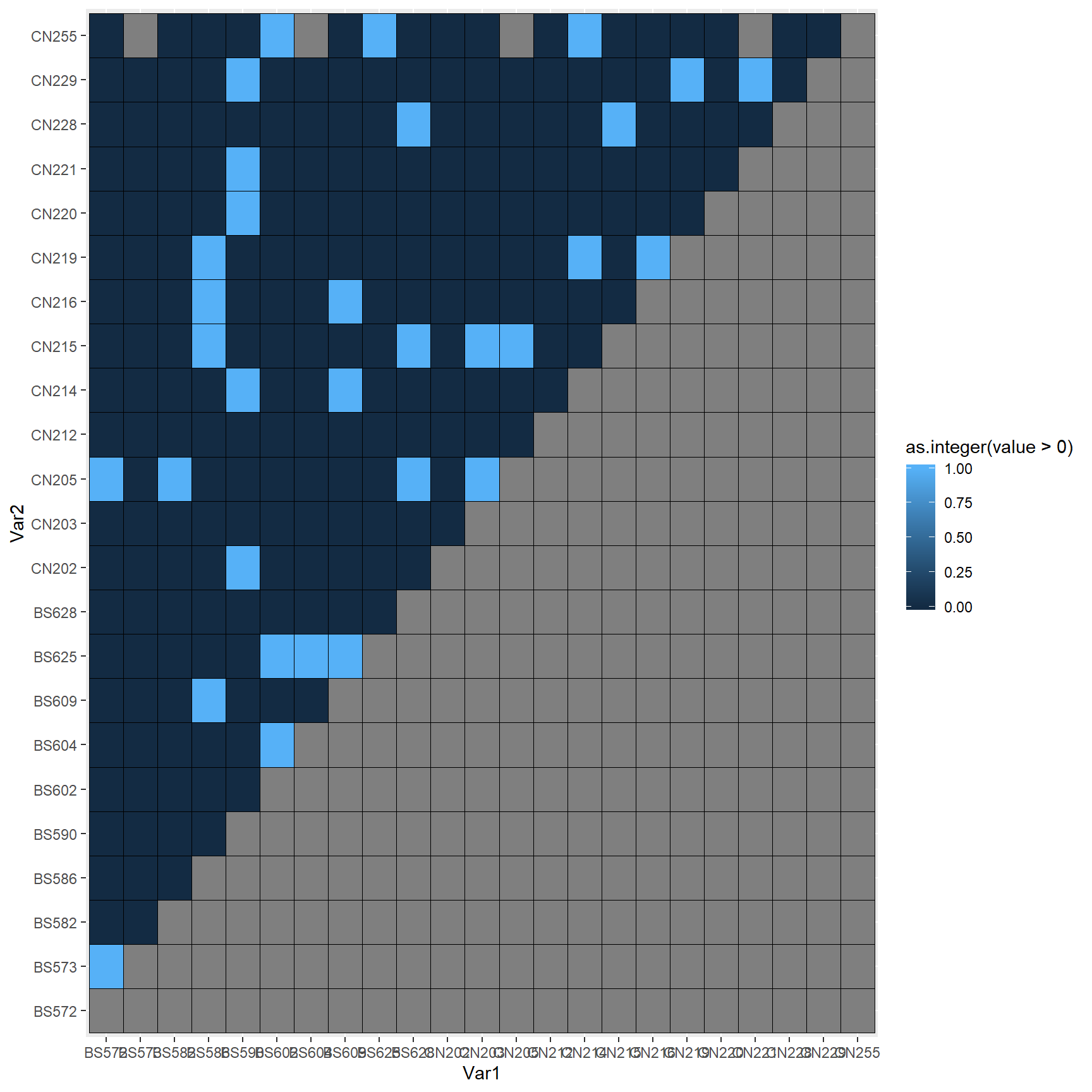#make a graph based on the "edges" we've identified
n_close_g1 = igraph::graph_from_data_frame(n_close_df_nz, directed = FALSE)
plot(n_close_g1, vertex.size = 11, vertex.label.cex = .65)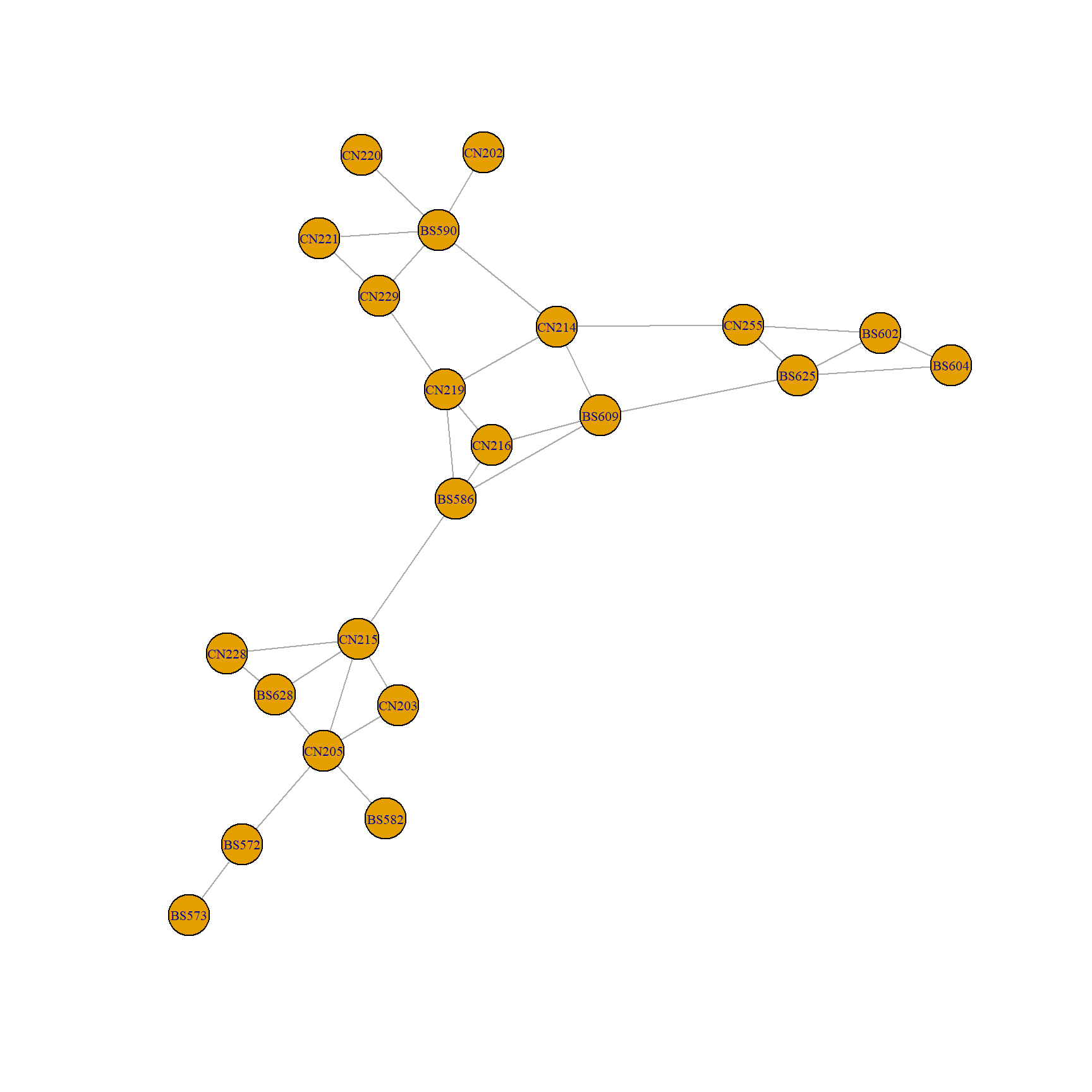This tells us which tortoises interacted, but if we were to add edge and node attributes we could make a more informative graph

We’ll make another graph - for this one we’ll pass in a data frame that gives attributes for the vertices. I want to include the centroids of each tortoise’s points - this will let us see how the relationships are arranged in space.

#find the centroids and then merge them with the tortoise info that we already have
tort_centroids = aggregate(cbind(x,y) ~ tort, torts, mean)
tort_info_xy = merge(tort_info, tort_centroids, by.x = "Tortoise", by.y = "tort")

#create another graph, this time providing attribute data for the vertices
n_close_g2 = igraph::graph_from_data_frame(n_close_df_nz, directed = FALSE, vertices = tort_info_xy)

#one way to adjust plot parameters is to assign attributes with certain names to the edges or vertices - these will be used when plotting
#we'll make the edge width vary based on how many contacts the tortoises had (thicker means more)
E(n_close_g2)$width = adj_vals(log(E(n_close_g2)$value), new_min = 1, new_max = 15) #take the log of the values because the data is skewed to the right
#we'll color the vertices according to sex
tort_cols = c("M" = "cornflowerblue", "F" = "lightcoral")
V(n_close_g2)$color = tort_cols[V(n_close_g2)$LikelySEX]

#because we have vertex attributes of x and y, it will automatically put the vertices at those coordinates
plot(n_close_g2,
vertex.size = 11,
vertex.label.cex = .65)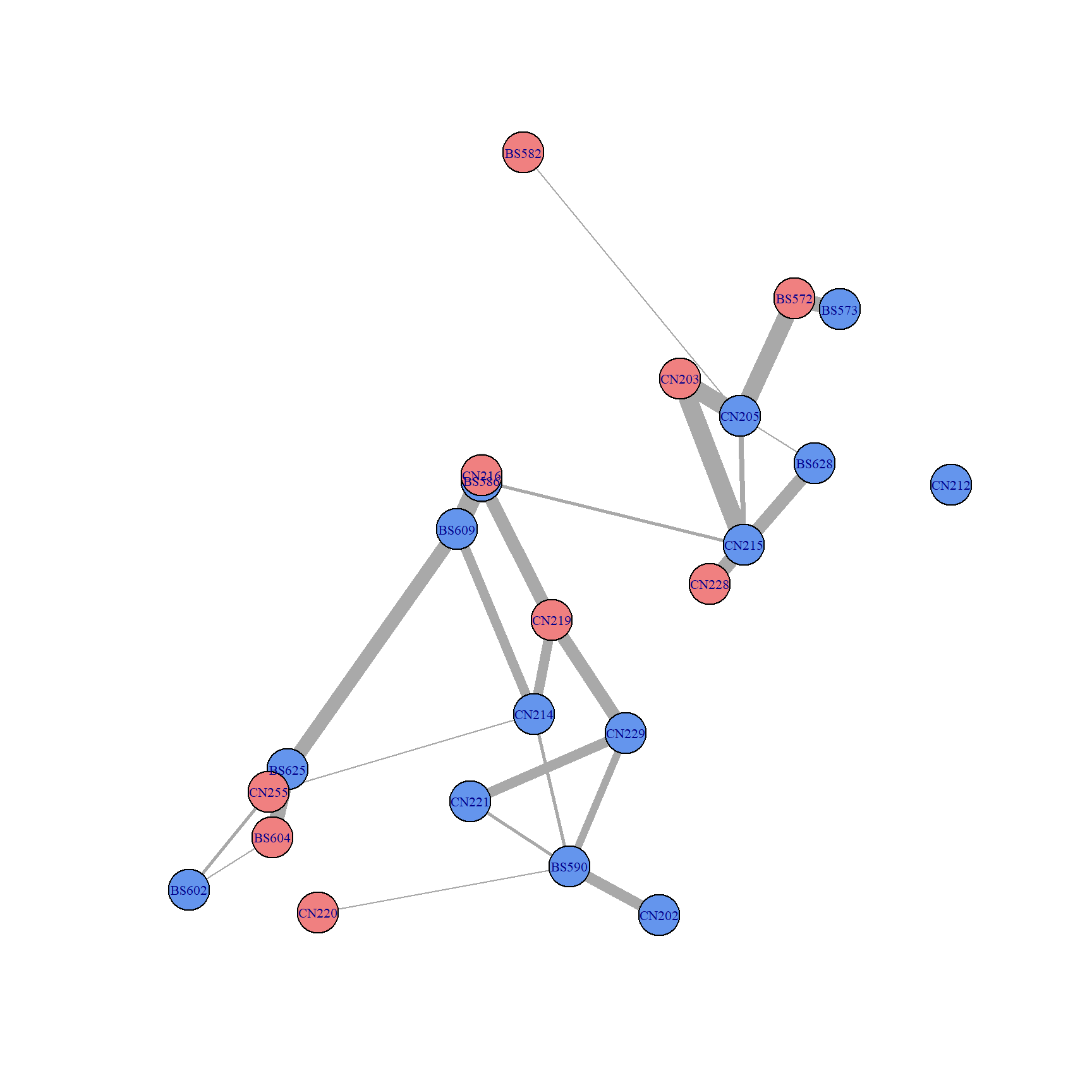If we don’t want it to plot it spatially, we can set the layout argument to be the function layout_with_fr(graph_name).

plot(n_close_g2,
vertex.size = 11,
vertex.label.cex = .65,
layout = layout_with_fr(n_close_g2))